Ex 7.2

Chapter 7 Class 8 Comparing Quantities
Serial order wise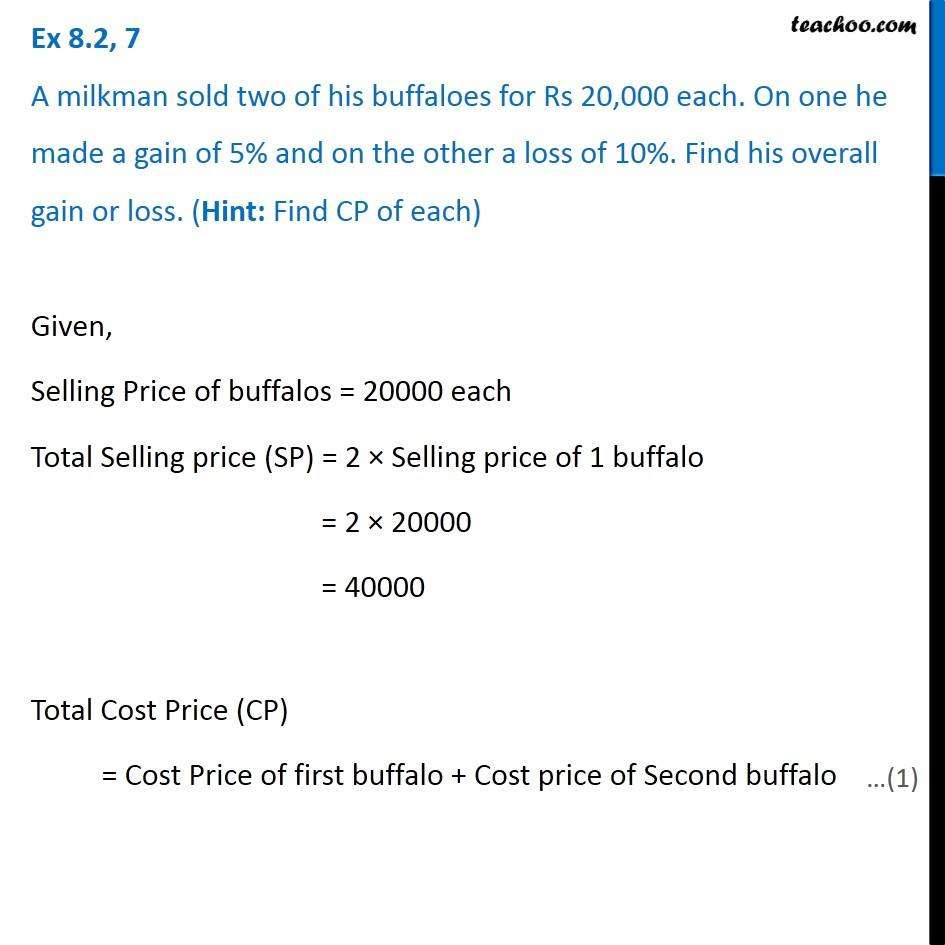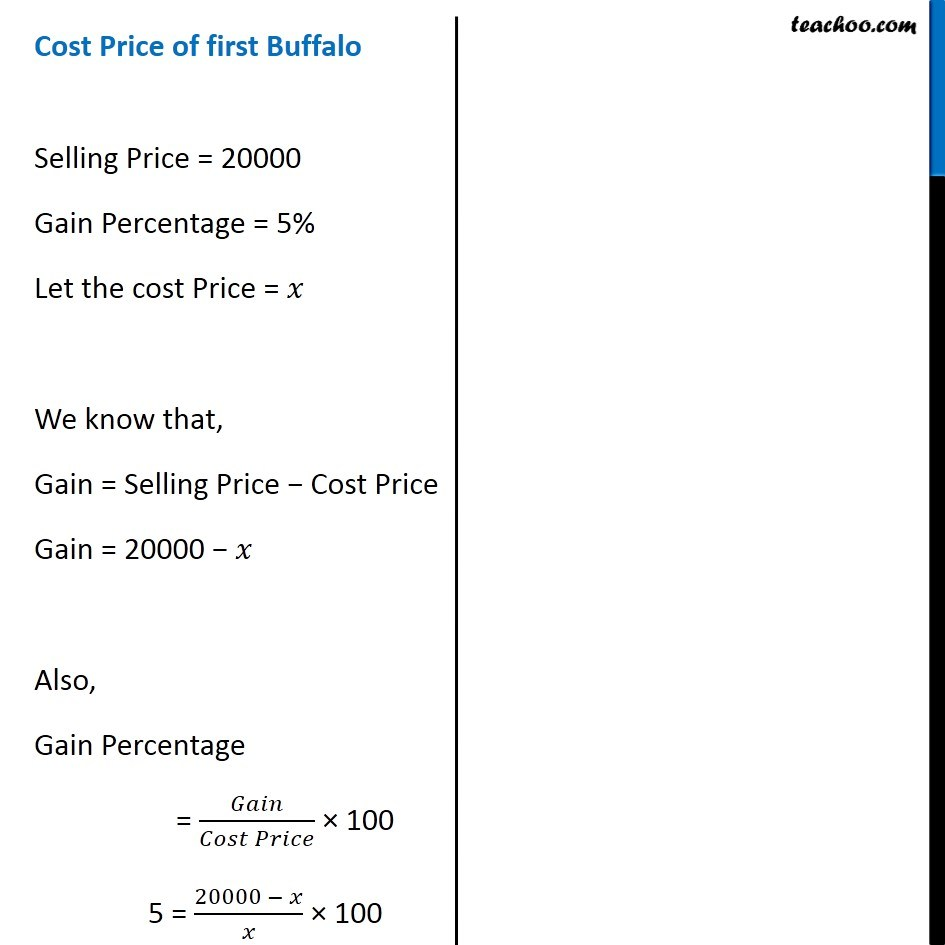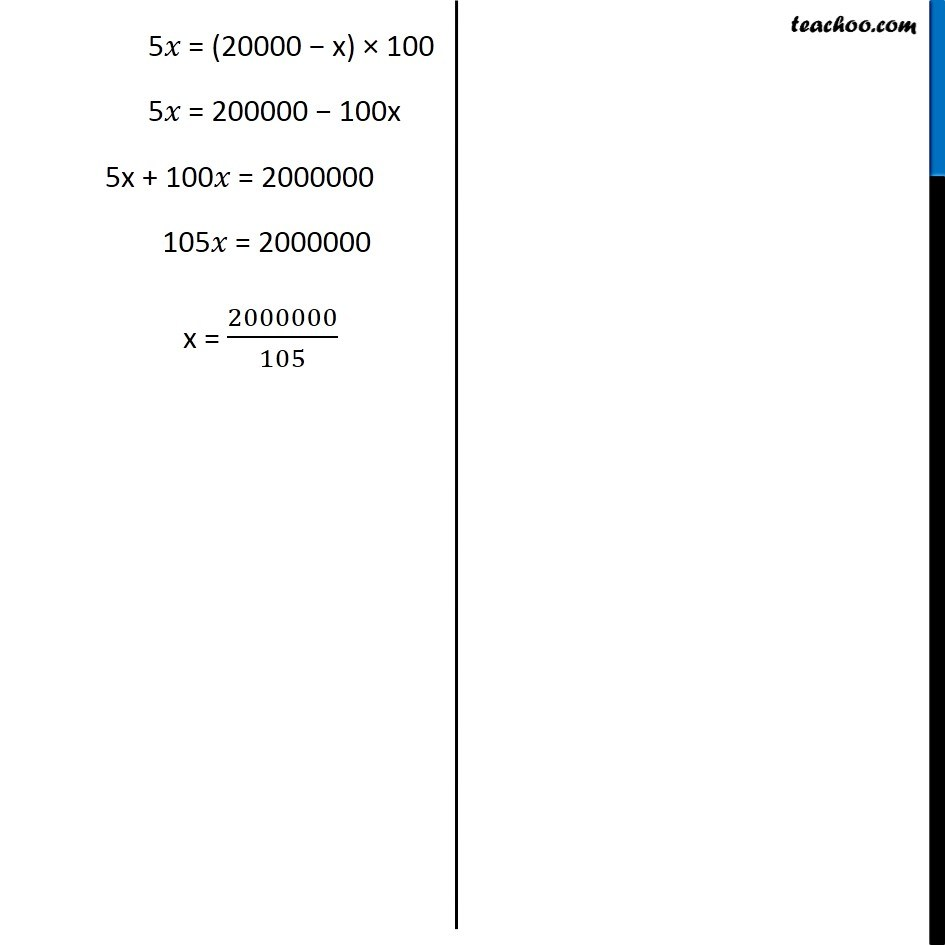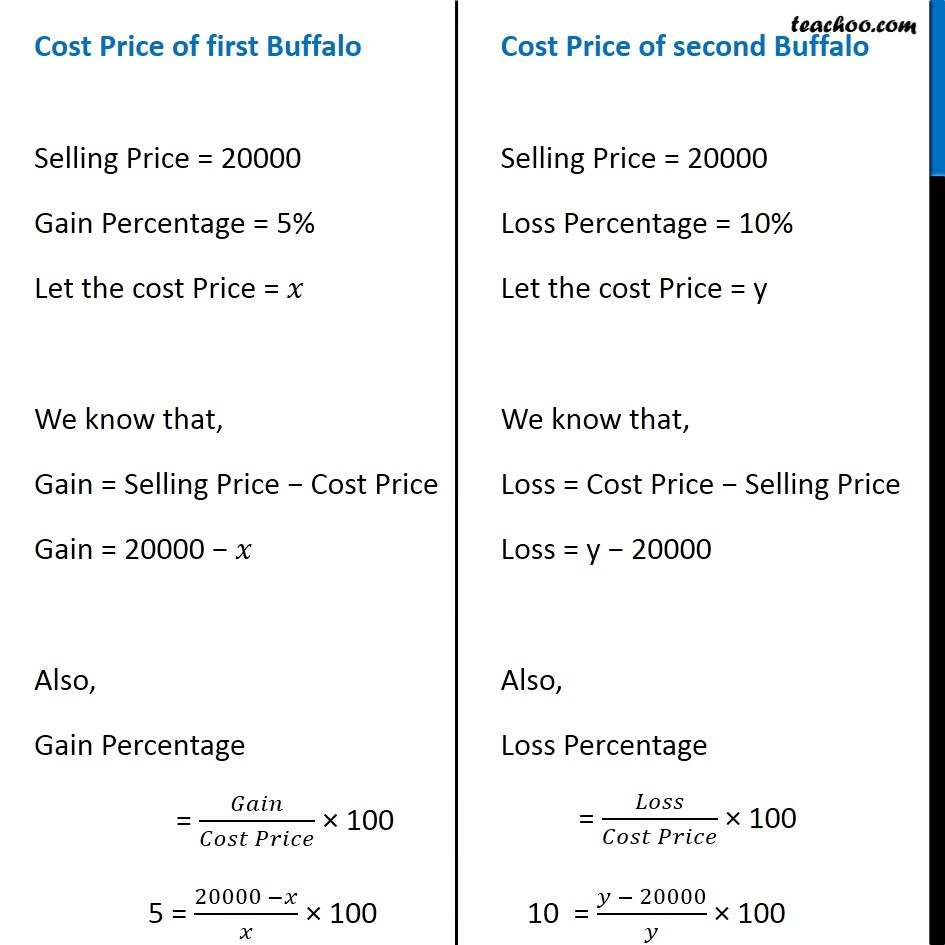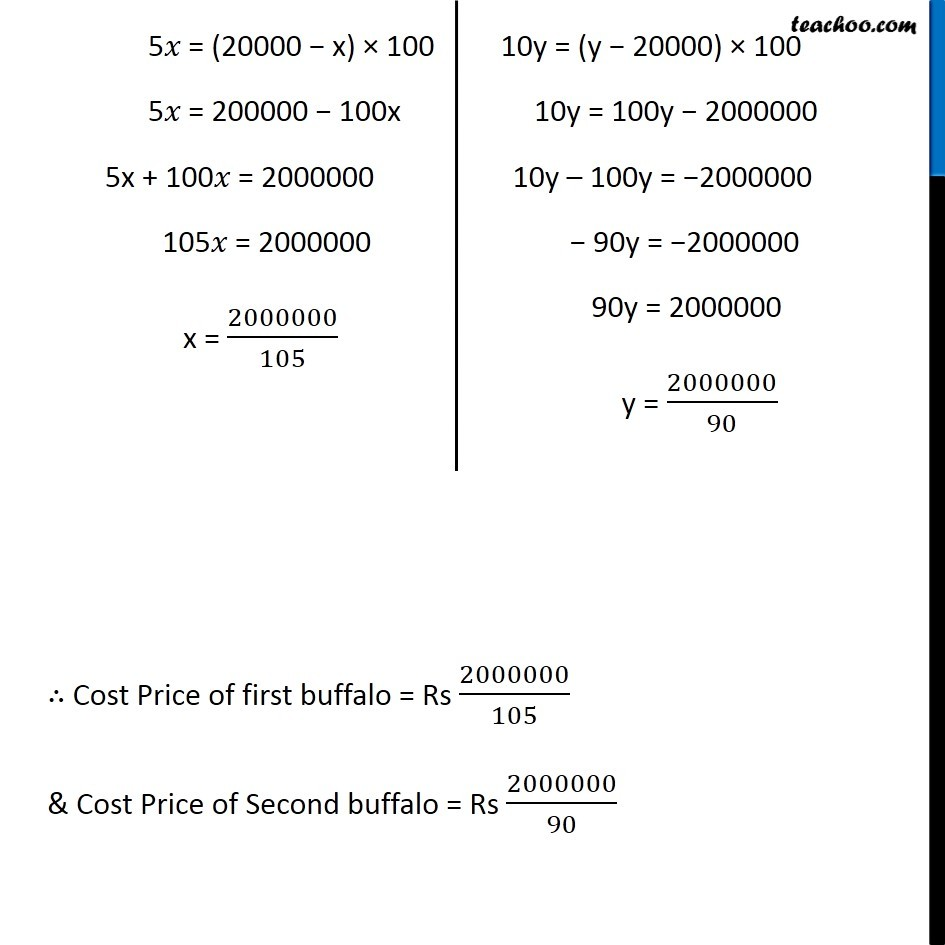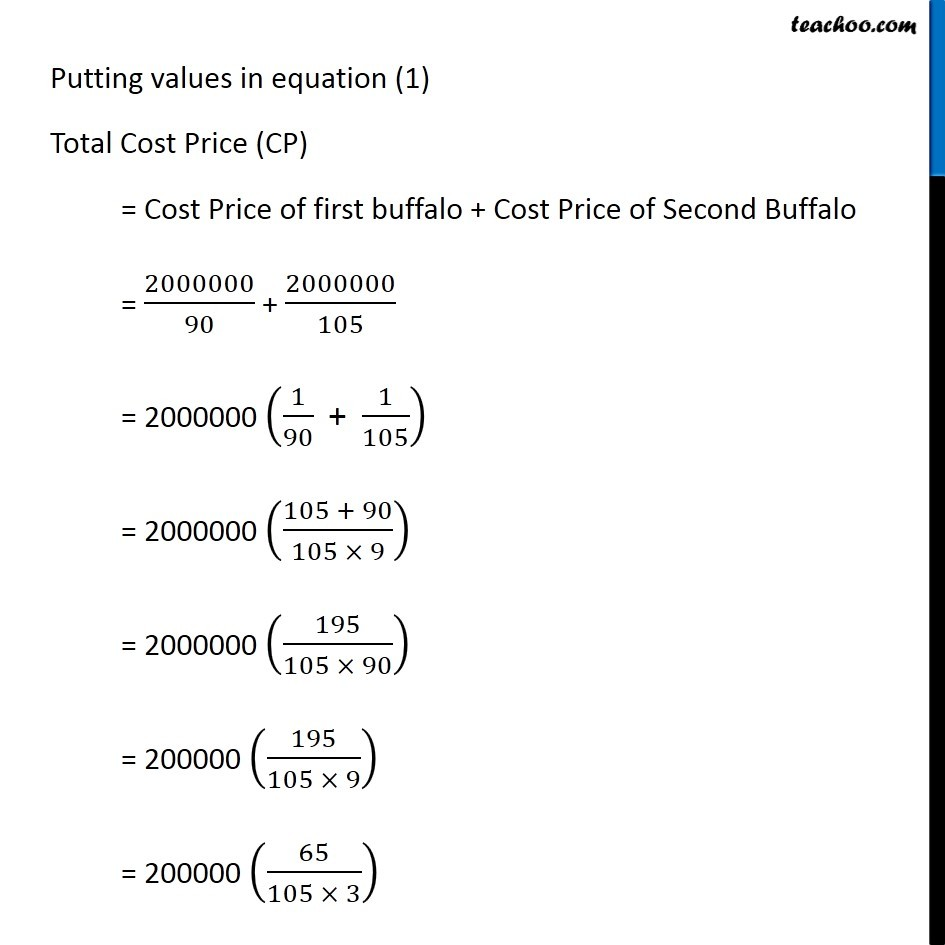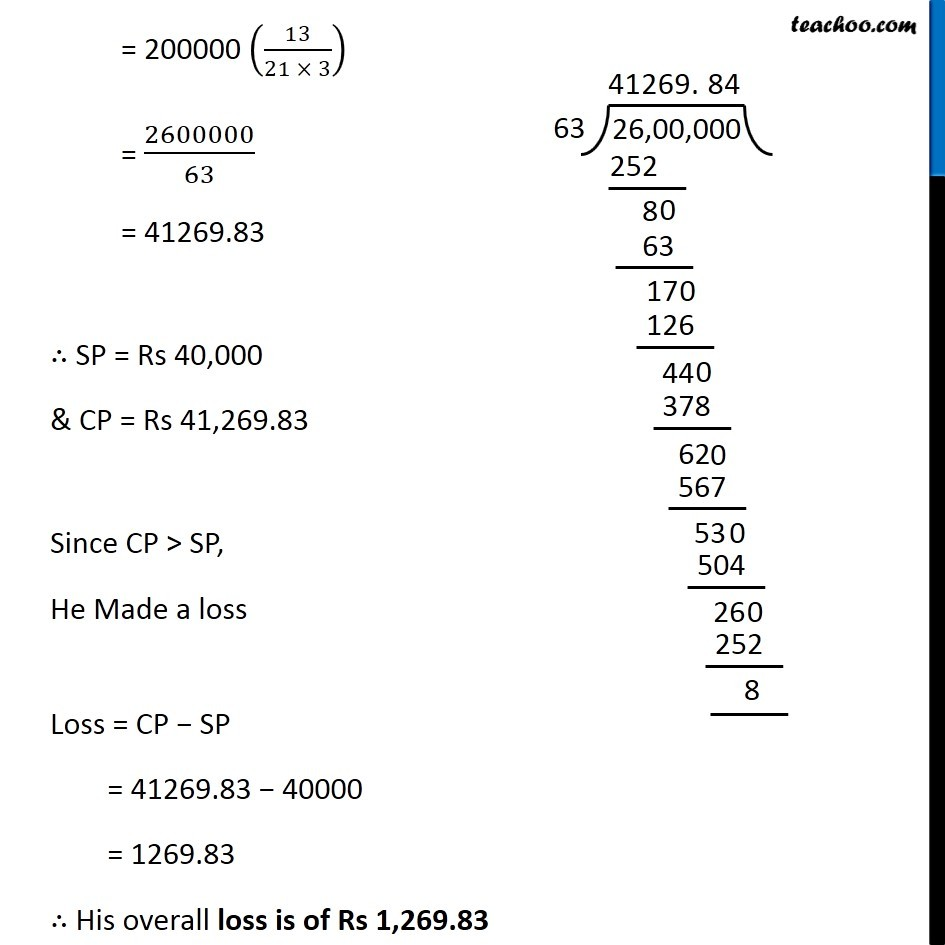Learn in your speed, with individual attention - Teachoo Maths 1-on-1 Class

### Transcript

Question 6 A milkman sold two of his buffaloes for Rs 20,000 each. On one he made a gain of 5% and on the other a loss of 10%. Find his overall gain or loss. (Hint: Find CP of each) Given, Selling Price of buffalos = 20000 each Total Selling price (SP) = 2 × Selling price of 1 buffalo = 2 × 20000 = 40000 Total Cost Price (CP) = Cost Price of first buffalo + Cost price of Second buffalo Cost Price of first Buffalo Selling Price = 20000 Gain Percentage = 5% Let the cost Price = 𝑥 We know that, Gain = Selling Price − Cost Price Gain = 20000 − 𝑥 Also, Gain Percentage = 𝐺𝑎𝑖𝑛/(𝐶𝑜𝑠𝑡 𝑃𝑟𝑖𝑐𝑒) × 100 5 = (20000 − 𝑥)/𝑥 × 100 5𝑥 = (20000 − x) × 100 5𝑥 = 200000 − 100x 5x + 100𝑥 = 2000000 105𝑥 = 2000000 x = 2000000/105 Cost Price of first Buffalo Selling Price = 20000 Gain Percentage = 5% Let the cost Price = 𝑥 We know that, Gain = Selling Price − Cost Price Gain = 20000 − 𝑥 Also, Gain Percentage = 𝐺𝑎𝑖𝑛/(𝐶𝑜𝑠𝑡 𝑃𝑟𝑖𝑐𝑒) × 100 5 = (20000 −𝑥)/𝑥 × 100 Cost Price of second Buffalo Selling Price = 20000 Loss Percentage = 10% Let the cost Price = y We know that, Loss = Cost Price − Selling Price Loss = y − 20000 Also, Loss Percentage = 𝐿𝑜𝑠𝑠/(𝐶𝑜𝑠𝑡 𝑃𝑟𝑖𝑐𝑒) × 100 10 = (𝑦 − 20000)/𝑦 × 100 5𝑥 = (20000 − x) × 100 5𝑥 = 200000 − 100x 5x + 100𝑥 = 2000000 105𝑥 = 2000000 x = 2000000/105 10y = (y − 20000) × 100 10y = 100y − 2000000 10y – 100y = −2000000 − 90y = −2000000 90y = 2000000 y = 2000000/90 ∴ Cost Price of first buffalo = Rs 2000000/105 & Cost Price of Second buffalo = Rs 2000000/90 Putting values in equation (1) Total Cost Price (CP) = Cost Price of first buffalo + Cost Price of Second Buffalo = 2000000/90 + 2000000/105 = 2000000 (1/90 " + " 1/105) = 2000000 ((105 + 90)/(105 × 9)) = 2000000 (195/(105 × 90)) = 200000 (195/(105 × 9)) = 200000 (65/(105 × 3)) = 200000 (13/(21 × 3)) = 2600000/63 = 41269.83 ∴ SP = Rs 40,000 & CP = Rs 41,269.83 Since CP > SP, He Made a loss Loss = CP − SP = 41269.83 − 40000 = 1269.83 ∴ His overall loss is of Rs 1,269.83